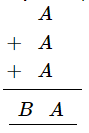# Replace A, B, by suitable numerals.

Question:

Replace A, B, by suitable numerals.Solution:

$A+A+A=A \quad$ (with 1 being carried over)

This is satisfied if $A$ is equal to 5 .

When $A=5$ :

$A+A+A=15$ ( 1 is carried over)

Or $B=1$

$\therefore A=5$ and $B=1$